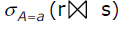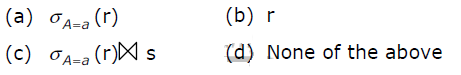Open in App
Not now

# GATE | GATE-CS-2001 | Question 25

• Last Updated : 28 Jun, 2021

Let r and s be two relations over the relation schemes R and S respectively, and let A be an attribute in R. then the relational algebra expressionis always equal to(A) A
(B) B
(C) C
(D) D

Explanation: The above expression evaluates A = a for tables r and s

Option A : is only displaying attributes of table r on the select condition

Option B : is only displaying attributes of table r

Option C: evaluates A = a by joining tables r and s  efficiently , thus correct